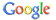web uni-protokolle.de powered by# Chaos and Chance. An Indroduction to Stochastic Aspects of Dynamics.von Arno BergerKategorie: StochastikISBN: 3110169908 Kommentar abgeben

Synopsis With emphasis on stochastic aspects of deterministic systems this short book introduces the reader to the basic facts and some special topics of applied ergodic theory. It adresses advanced undergraduate and graduate students from various disciplines, i.e. mathematicians, physicists, electrical and mechanical engineers. Based upon a sound (but non-technical) mathematical introduction, a number of typical examples from applications (mostly from mechanics) are thoroughly discussed. By studying both probabilistic and deterministic features of dynamical systems the reader will develop what might be considered a unified view on chaos and chance as two sides of the same thing.

 Stochastik > Chaos and Chance. An Indroduction to Stochastic Aspects of Dynamics.

HTML-Code zum Verweis auf diese Seite:
<a href="http://www.uni-protokolle.de/buecher/isbn/3110169908/">Chaos and Chance. An Indroduction to Stochastic Aspects of Dynamics. </a>